Next: C Solutions to Schrödinger's Up: Dissertation Christoph Wasshuber Previous: A.4 Capacitance of an

# B Fermi Energy Dependence on Free Charge Carrier Concentration

The number of allowed k-values per unit volume of k-space in a piece of material with volume V is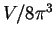. The number of energy levels in the Fermi sphere, considering two spin values for each k-value, is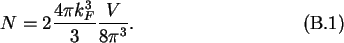Thus if there are N electrons in a volume V the concentration is n = N/V, which leads to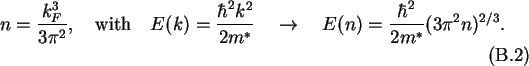In the case of semiconductors the band gap has to be taken into account. Using the  Boltzmann approximation to the Fermi distribution the carrier concentrations in the conduction and valence band are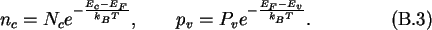Noting that for the intrinsic case, EF = EF,i, nc=pv=ni the concentrations may be written as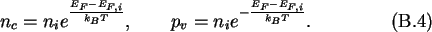And with the net carrier concentration n=nc-pv one derives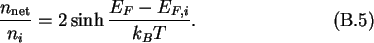Christoph Wasshuber